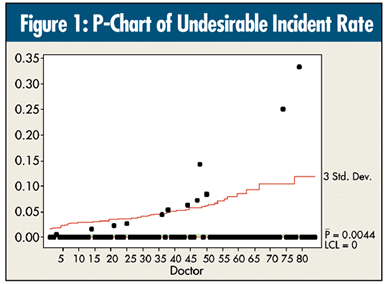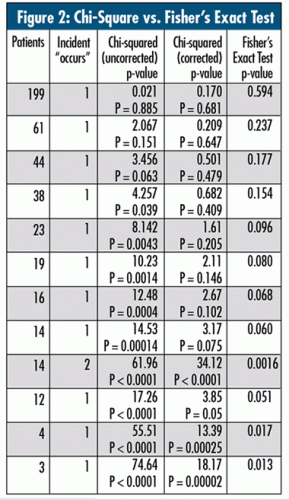# Quality DigestBio

## Analyzing Rare Occurrences of Events

### When to use Fisher’s Exact Test

Eighty-four doctors treated 2,973 patients, and an undesirable incident occurred in 13 of the treatments (11 doctors with one incident and one doctor with two incidents), a rate of 0.437 percent. A p-chart analysis of means (ANOM) for these data is shown in figure 1.This analysis is dubious. A good rule of thumb: Multiplying the overall average rate by the number of cases for an individual should yield the possibility of at least five cases. Each doctor would need 1,000 cases to even begin to come close to this!

The table in figure 2 uses the technique discussed in last month’s column, “A Handy Technique to Have in Your Back Pocket,” calculating both “uncorrected” and “corrected” chi-square. Similar to the philosophy of ANOM, I take each doctor’s performance out of the aggregate and compare it to those remaining to see whether they are statistically different. For example, in figure 2, during the first doctor’s performance, one patient in the 199 patient treatments had the incident occur. So, I compared his rate of 1/199 to the remaining 12/2,774.Things break down very quickly as the denominator size decreases, especially the gap between the “uncorrected” and “corrected” chi-square values.

With data like these, one has no option but to use the technique known as Fisher’s Exact Test (available in most good statistical packages). Its resulting p-value is shown in the far right column of figure 2. Using the example of the doctor with one incident out of 199 patients, one has to ask, “If I have a population where 13 out of 2,973 patients experienced an incident, and if I grabbed a random sample of 199 of these 2,973 patients, what is the probability that I would have at least one patient who had an incident?” As you can see in figure 2, in the first row of the Fisher’s exact test column, it is 0.594 (~ 60%)--not unusual.

Figure 3 sets up the calculation for the only doctor for whom two patients had the event occur (out of 14 patients). So, one is comparing 2/14 vs. 11/2,959. One now has to calculate the exact probabilities of randomly obtaining zero (p 0) and one (p 1) event in a random sample of 14, then calculating (1 - (p 0 + p 1)) to answer, “What is the probability of obtaining two or more events in this sample due to sheer randomness?” As you see from the table in figure 2, it is 0.0016 (~0.2%).The question now becomes, “What constitutes an outlier?” To put things in perspective, I’m going to use the technique discussed in my February 2006 column, “Why Three Standard Deviations?” to see what the threshold of probability might be for overall risks of 0.05 and 0.10 (one-tailed).

In this case of 84 simultaneous decisions:

Overall 5-percent risk - p < 0.00061 to declare “significance”

Overall 10-percent risk - p < 0.00125

Only the 2/14 is close when compared with these criteria, but barely at the 10-percent risk level.

There are never any easy answers when rates of rare adverse events regarding human life are being compared and someone’s professional reputation is at stake. At least choose the correct analysis, and beware of packaged “easy answers.”

### Discuss